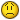Sum all items from the given year

R

Ricardo

I have two columns:
A - formated as Date ("YYYY-MM-DD") with dates of purchase
B - formated as Number with cost of purchase
The columns constantly grow or shrink as I am adding new items or deleting them.
I already figured out how to sum up the global cost of purchase without having to define a rigid range in the B column:
=SUM(\$B:\$B)
It works just fine for me summing up all of the non-empty cells in column B.
Now I would like to sum up the cost of purchase for a given year, eg. 2011.
It should probably look like:
=SUM.IF(\$A:\$A;"GIVEN YEAR";\$B:\$B)
However, I cannot figure out how to define the condition for "GIVEN YEAR"I

isabelle

hi Ricardo,

=SUMPRODUCT((YEAR(A2:A100)=2011)*(B2:B100))

R

Ricardo

Thanks a lot Isabelle. It worked like a charm!Would you please tell me how to count all purcheses for a given year?

I

isabelle

ok but i'm not sure if is that what you want

=SOMMEPROD((ANNEE(A3:A65536)=2011)*1)

=SOMMEPROD((ANNEE(A3:A65536)=2011)*(B3:B65536))

C

Cimjet

Isabelle
Les formules sont en francais.
Cimjet

I

isabelle

oups sorry,

=SUMPRODUCT((YEAR(A3:A65536)=2011)*1)

=SUMPRODUCT((YEAR(A3:A65536)=2011)*(B3:B65536))

R

Ricardo

Great! Thanks again Isabelle!!! You just made my day!!!Best wishes,
Ricardo